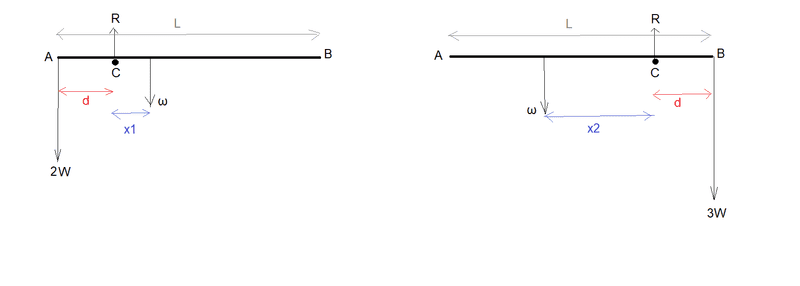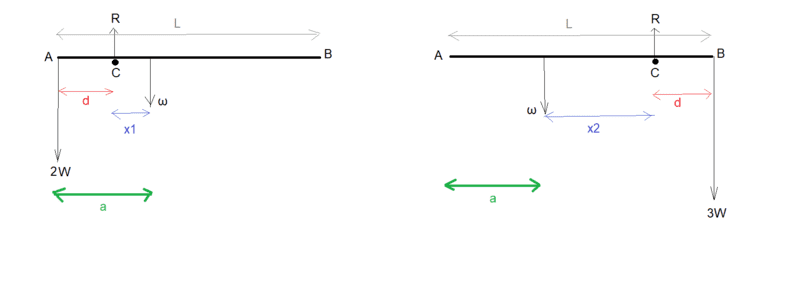# To locate the centre of gravity of a rod

gnits
Homework Statement:
To locate the centre of gravity of a rod
Relevant Equations:
moments
Could I please ask for a help on how to attack this question?

A heavy rod AB of length L can be made to balance across a small smooth peg C when a weight of 2W is suspended from A. Alternatively, it can be made to balance across the peg with a weight of 3W suspended from B. If the distance AC in the first case is the same as the distance BC in the second, show that the distance of the centre of gravity of the rod from A lies between (2/5)L and (1/2)L.

Here is my diagram for the two cases.(I have called the weight of the rod ω)

In each diagram I have labelled ω as acting at the same point, but not at the centre point of AB, as I take it from this question that the rod is not of uniform density.

Well, if I take moments about C in the first case I get:

2Wd=ω*x1 giving x1 = 2Wd/ω

So the distance of the centre of gravity of the rod from A is:

d + x1 = d + 2Wd/ω.

Well, I can do the same for the second case giving distance of centre of gravity of the rod from A as:

L - d - x2 = L - d - 3Wd/ω

Thanks for any pointer towards a solution,
Mitch.

•Delta2

Homework Helper
Gold Member
The problem is telling you that the actual center of mass of the rod is located between 40% and 50% of L, when measured from end A.

Homework Helper
Gold Member
2022 Award
Homework Statement:: To locate the centre of gravity of a rod
Relevant Equations:: moments

Could I please ask for a help on how to attack this question?

A heavy rod AB of length L can be made to balance across a small smooth peg C when a weight of 2W is suspended from A. Alternatively, it can be made to balance across the peg with a weight of 3W suspended from B. If the distance AC in the first case is the same as the distance BC in the second, show that the distance of the centre of gravity of the rod from A lies between (2/5)L and (1/2)L.

Here is my diagram for the two cases.

View attachment 293869
(I have called the weight of the rod ω)

In each diagram I have labelled ω as acting at the same point, but not at the centre point of AB, as I take it from this question that the rod is not of uniform density.

Well, if I take moments about C in the first case I get:

2Wd=ω*x1 giving x1 = 2Wd/ω

So the distance of the centre of gravity of the rod from A is:

d + x1 = d + 2Wd/ω.

Well, I can do the same for the second case giving distance of centre of gravity of the rod from A as:

L - d - x2 = L - d - 3Wd/ω

Thanks for any pointer towards a solution,
Mitch.
It's a tricky problem. A useful idea is the introduce the dimensionless constant ##\mu = \frac{W}{w}##, which is the ratio of the weight of the rod and the the other unknown weight ##W##.

The position of the CoM of the rod must depend on this ratio.

The variable you must eliminate is ##d##, leaving the distance from A to the CoM in terms of ##L## and ##\mu##.

I also suggest you need a symbol for this distance from A to the CoM. E.g. ##a##.

•Delta2
gnits
It's a tricky problem. A useful idea is the introduce the dimensionless constant ##\mu = \frac{W}{w}##, which is the ratio of the weight of the rod and the the other unknown weight ##W##.

The position of the CoM of the rod must depend on this ratio.

The variable you must eliminate is ##d##, leaving the distance from A to the CoM in terms of ##L## and ##\mu##.

I also suggest you need a symbol for this distance from A to the CoM. E.g. ##a##.
Thank you very much for your reply. I would never have thought of that approach. So here is my previous diagram, with 'a' added:I can combine my previously derived equations with your suggested terminology to give:

(am using u for the symbol 'mu')

a = d(1 + 2u)

and

a = L - d(1 + 3u)

and I eliminate d from these to give:

a = L - a * (1+3u)/(1+2u)

(Call this "Equation 1")

and can arrange this for a like so:

a = L(1 + 2u)/(2 + 5u)

(Call this "Equation 2")

So, tantalizingly, I can see that from "Equation 2" 'a' is not defined when u = -2/5 and from "Equation 1" 'a' is not defined when u = -1/2

I can't yet though see how to relate this to the required answer - required answer being that the distance of COG from A >(2/5)L and <(1/2)L

EDIT:
OK so looking at "Equation 2". I need 0 < a < L so I need:

0 < (1 + 2u)/(2 + 5u) < 1

Solving 0 < (1 + 2u)/(2 + 5u) leads to:

-1/2 > u > -2/5

and solving (1 + 2u)/(2 + 5u) < 1 leads to:

-2/5 > u > -1/3

Remembering that u = W/ω I can't yet see how to get from these limits on W/ω to limits for distance of COG from A.

I'll keep looking,
Thanks for any further help,
Mitch.

Last edited:
•PeroK
Homework Helper
Gold Member
2022 Award
Thank you very much for your reply. I would never have thought of that approach. So here is my previous diagram, with 'a' added:View attachment 293930
I can combine my previously derived equations with your suggested terminology to give:

(am using u for the symbol 'mu')

a = d(1 + 2u)

and

a = L - d(1 + 3u)

and I eliminate d from these to give:

a = L - a * (1+3u)/(1+2u)

(Call this "Equation 1")

and can arrange this for a like so:

a = L(1 + 2u)/(2 + 5u)

(Call this "Equation 2")
Once you have equation 2 you are nearly finished. ##u = \frac{W}{w}## is the ratio of the weights and can take any positive value. You only need to calculate the maximum and minimum for ##a##.

So, tantalizingly, I can see that from "Equation 2" 'a' is not defined when u = -2/5 and from "Equation 1" 'a' is not defined when u = -1/2
##u## cannot be negative as it's a ratio of weights/masses.

•Lnewqban
gnits
Once you have equation 2 you are nearly finished. ##u = \frac{W}{w}## is the ratio of the weights and can take any positive value. You only need to calculate the maximum and minimum for ##a##.

##u## cannot be negative as it's a ratio of weights/masses.

I see it!

I would never have gotten it without your help. In particular, setting the equations in terms of W/ω, I don't see how I would ever of thought of that.

Thank you very much indeed.

Mitch.

•